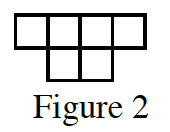### Home > CC4 > Chapter 5 > Lesson 5.3.3 > Problem5-125

5-125.

Figure $2$ of a tile pattern is shown at right.  If the pattern grows linearly and if Figure $5$ has $15$ tiles, then find a rule for the pattern. Homework Help ✎

Make a table relating the figure number to the number of tiles, or write a proportion.

$y=3x$